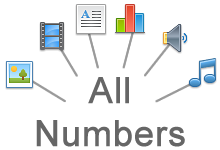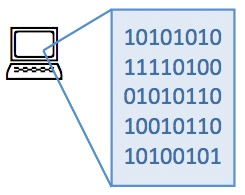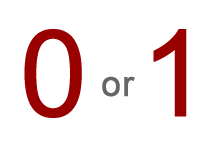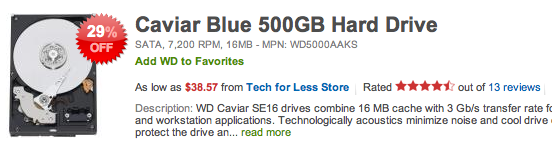Note: You do not need to have a deep understanding of the information on this page. However it may well help you understand some of the other theory topics, and also may help explain some of the computer terms that you may have heard, such as MegaByte.

## How is Data Actually Stored in a Computer?

Everything that is stored and processed inside a computer (all data, information, instructions, files, images, etc.) is stored as numbers. This is because computer only understands numbers, nothing else.

Numbers are made up of digits, so we could say that computers only understand digits. Because of this, we say that computers are digital devices.This sounds a bit strange: how do you store text and pictures as numbers?!

The solution is to use numeric codes:
• Different letters in a text document are given different numeric codes
• Different pixels (coloured dots) in an image are given different numeric codes
• Different sounds in a music file are given different numeric codes
Everything is numbers!
The numeric codes used for letters are defined by a system called the American Standard Code for Information Interchange (ASCII).

For example, the letter ‘A’ has the ASCII code 65.
More specifically, everything inside a computer is actually a Binary number (see the explanation to the right).

If you took a peek inside the memory of a computer you might see the following Binary numbers:

10100111
11010101
00101001These numbers could be the codes for some text, or they could be the codes for some pixels in an image, or they could just be numbers!
A computer only understands a particular type of number called Binary.

This rather strange number system (and you really don't need to learn this!) only uses the digits 0 and 1.

So a typical binary number might be 011101010.

The reason for the use of binary numbers is that the electronic devices in a computer (the CPU, the RAM, etc.) have parts that can only be turned on or off. We can use binary numbers to control these ons and offs: off = 0, on = 1

## What is a Bit?

A bit is a Binary Digit (the word comes from Binary DigIT)

Because it is a binary digit, a bit can only have the value 0 or 1. (This is different from our normal decimal digits which can have any value between 0 and 9.)

This binary number is four bits long: 1101## What is a Byte?

Bits are usually grouped into groups of 8, and each group is called a Byte.

Here is are a bunch of bytes (all exactly 8 bits long):

10101011
01110010
10101010
00001111

Bytes are the standard unit of storage for all computing devices: Hard drive size is measured in bytes, file size is measured in bytes, RAM size is measured in bytes.

So, next time someone tells you that they have a 500 GB hard drive, you will know that it can store 500 billion bytes of data.In the example above, the hard drive:
• can store 500GB of data (500 billion bytes)
• has a cache of 16MB (16 million bytes)
• can transfer data at a rate of 3Gb/s (3 billion bits per second)
Counting Bytes

A thousand bytes is known as a kilobyte (kB)
1,000B = 1kB

A million bytes is known as a megabyte (MB)

1,000,000B = 1MB

A thousand million bytes is called a gigabyte (GB)

1,000,000,000B = 1GB

A million million bytes is called a terabyte (TB)

1,000,000,000,000B = 1TB
The unit 'bit' is normally abbreviated to a lowercase 'b', and the unit 'byte' is abbreviated to an uppercase 'B'.

So 512 kilobits is written 512kb and 500 megabytes is written 500MB
Because you know that there are 8 bits in a byte, you'll also have worked out that the hard drive can store 500 x 8 = 4000 billion bits.

That's 4,000,000,000,000 bits.

That's a lot of 0s and 1s!

#### Sorry to ask you this, but...

This site took quite a bit of time and effort to create, and it costs me money to keep it up and running. If the site has helped you, please consider showing your appreciation by donating a little towards the site's running costs.

Thank you!